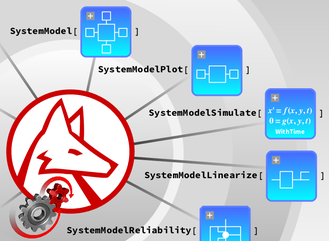# System Modeling

Version 12 includes full system modeling and analysis capability in the Wolfram Language. System models are typically used to understand and optimize engineering design in areas such as electrical, mechanical, thermal and many more. System models describe complex systems with high fidelity and give accurate results, shortening the design cycle in all modern engineering projects.• Use any of 500 prebuilt example models. »
• Create models by using 7000+ components in multiple engineering domains. »
• Create models from equations and combine them with existing components. »
• Create models using the SystemModeler graphical user interface and directly use it in the Wolfram Language. »
• Simulate realistic models with thousands of variables. »
• Simulate under parameter variations in parallel. »
• Simulate parameter sensitivity of any model. »
• Directly plot any of thousands of possible model variables. »
• Automatically create linearized models for control design. »
• Calibrate model parameters from data.
• Optimize system performance.
• Combine dynamic simulation and reliability analysis. »